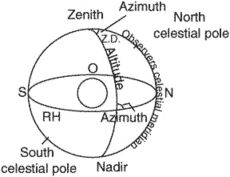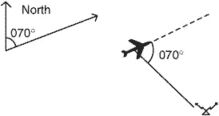# azimuth angle

Also found in: Dictionary, Acronyms.
Related to azimuth angle: Zenith Angle

## azimuth angle

[′az·ə·məth ′aŋ·gəl]
(engineering)
An angle in triangulation or in traverse through which the computation of azimuth is carried.
McGraw-Hill Dictionary of Scientific & Technical Terms, 6E, Copyright © 2003 by The McGraw-Hill Companies, Inc.

## azimuth angle (Z)Azimuth angles in both these two cases are 070°.
i. The arc of the horizon between the observer's meridian and the vertical circle of an object, measured either from the north or south, to the right or clock-wise, or to the left or counterclock-wise, through 90° or 180°. It must be labeled north or south (as a prefix) and east or west (as a prefix) to indicate the direction of measurement.
ii. An angle measured clock-wise in the horizontal plane between a reference direction and any other line.
References in periodicals archive ?
The wind factor and its interactions showed insignificant impact on the response except the interaction with the azimuth angle (orientation) of the PV panel.
CBF is the most traditional and the most classic technique used to estimate azimuth angle of an acoustic source.
Since the azimuth angle is directly observable by VO, shown by the design matrix [H.sub.VO] in (6), the system retains convergence even during intermittent GPS availability.
where [[theta].sub.s] is the squint angle of the monopulse beam, [[theta].sub.el] and [[theta].sub.az] are the differences of elevation angles and azimuth angles between the tracking axis and target, respectively, and [[theta].sub.A] is the angle difference between the beam maximum of quadrant 1 and target.
Specially, the convex pattern around the azimuth angles of 180 deg in the measured flap motion as well as an up-down pattern seen in the second quadrant of the measured torsion is captured nicely with the CFD/CSD coupling.
The estimation of azimuth angle via ITD is also given in (20).
Predetermined parameters are the zenith angle of the measurement a, the azimuth angle [beta], the field of view [phi], and the cloud-base height above the ground Az.
The geometry of our TIIR problem can be described as illustrated in Figure 1 where the tree-body is circularly scanned by a directive antenna for a total of M distinct look-aspects covering the whole azimuth angles. The radius of the scanning circle is [R.sub.0].
However, although the CNR of azimuth difference channel is far higher than the one of the elevation channel, the differences of the performance between the elevation and azimuth angle measurements are not obvious.
The distance between experimental specimen and target plates is 3.5 m, so the azimuth angle of each square region is 4.09[degrees].
The range of elevation angle is varied from 70[degrees] to 90[degrees] for fixed azimuth angle of 50[degrees].

Site: Follow: Share:
Open / Close